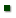Skip to main content
ARS Home » Research » Publications at this Location » Publication #166799

Title: VOLUNTARY FEED INTAKE BY LACTATING, ANGORA, GROWING, AND MATURE GOATS

AuthorLUO, JGOETSCH, ANSAHLAI, IMOORE, JGALYEAN, MJOHNSON, ZSAHLU, TFerrell, CalvinOWENS, F

 Submitted to: Meeting Proceedings Publication Type: Experiment Station Publication Acceptance Date: 3/5/2004 Publication Date: 4/2/2004 Citation: Luo, J., Goetsch, A.L., Nsahlai, I.V., Moore, J.E., Galyean, M.L., Johnson, Z.B., Sahlu, T., Ferrell, C.L., Owens, F.N. 2004. Voluntary feed intake by lactating, angora, growing, and mature goats. In: Proceedings of the 19th Annual Goat Field Day, April 24, 2004, Langston, Oklahoma, p. 151-153. Interpretive Summary: Technical Abstract: Because of the lack of methods to predict voluntary dry matter intake (DMI; kg/day) by goats, a database of treatment mean observations was constructed and used to describe DMI by lactating, Angora, growing, and mature goats. Predictions were based on calculated constant overall efficiencies of metabolizable energy (ME) utilization (k) based on ME requirements determined in companion studies and assumed efficiencies of ME use for different functions. For lactating goats, mean k was 0.653 (SE = 0.00134), and predicted DMI (P-DMI) was adjusted (AP-DMI) for the ratio of average daily gain (ADG, kg) to 4% fat-corrected milk (ADGFCM, kg/kg): AP-DMI (kg) = 0.0964 + (0.0334 x P-DMI) - (0.1237 x ADGFCM) (n = 191; R2 = 0.84). Mean k for Angora goats was 0.525 (SE = 0.0112), and prediction accuracy was improved by adjusting for dietary crude protein concentration (PTCP, %): AP-DMI (kg) = -0.1607 + (0.8227 x P-DMI) + (0.0199 x PTCP) (n = 54; R2 = 0.65). For growing goats, mean k was 0.634 (SE = 0.0020), and prediction accuracy was improved by adjusting for ratios of ADG to body weight (BW; ADGBW, kg/kg), kg body weight0.75 (MBW; ADGMBW, kg/kg0.75), and ADGMBW2: AP-DMI (kg) = -.0.0047 + (0.9637 x P-DMI) - (70.27 x ADGBW) + (38.71 x ADGMBW) - (243.4 x ADGMBW2) (n = 266; R2 = 0.88). Mean k for mature goats was 0.632 (SE = 0.00448), and prediction accuracy was improved by adjusting for PTCP (%), ADGBW (kg/kg), and ADGMBW (kg/kg0.75): AP-DMI (kg) = -0.1241 + (0.7915 x P-DMI) + (0.0214 x PTCP) - (535.2 x ADGBW) + (247.3 x ADGMBW) (n = 99; R2 = 0.85).